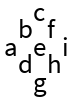#Function Repository Resource:

# Antidiagonal

Give the antidiagonal of a matrix

Contributed by: Sander Huisman
 ResourceFunction["Antidiagonal"][m] gives the list of elements on the leading antidiagonal of the matrix m. ResourceFunction["Antidiagonal"][m,k] gives the elements on the kth antidiagonal of m.

## Details and Options

An antidiagonal is defined as a list of elements for which the sum of the indices are equal, where the first element is in the top-right of a matrix: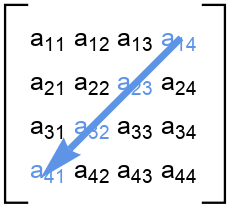ResourceFunction["Antidiagonal"][m] works even if m is not square.
For positive k, ResourceFunction["Antidiagonal"][m,k] gives antidiagonals above the leading antidiagonal. ResourceFunction["Antidiagonal"][m,-k] gives antidiagonals below.
ResourceFunction["Antidiagonal"] works on SparseArray matrices and will return a SparseArray list.

## Examples

### Basic Examples

Give the antidiagonal elements of a matrix:

 In:=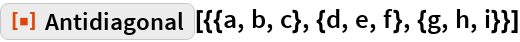Out=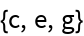Give antidiagonals one element above and below the leading diagonal:

 In:=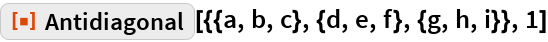Out=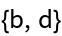In:=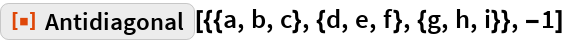Out=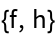Give an antidiagonal of a nonsquare matrix:

 In:=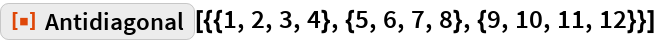Out=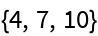### Properties and Relations

Antidiagonal and Diagonal are related to each other:

 In:=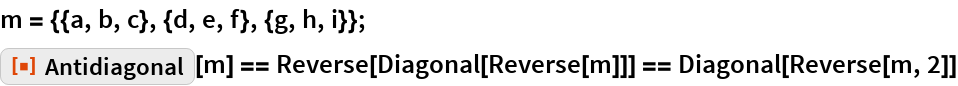Out=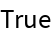### Possible Issues

If k is too high or too low, an empty list is returned:

 In:=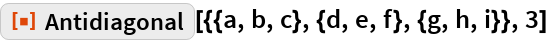Out=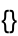### Neat Examples

Rotate a matrix by 45 degrees:

 In:=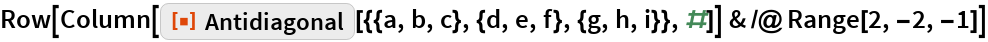Out=